# Adding and Subtracting Integers Lesson Plan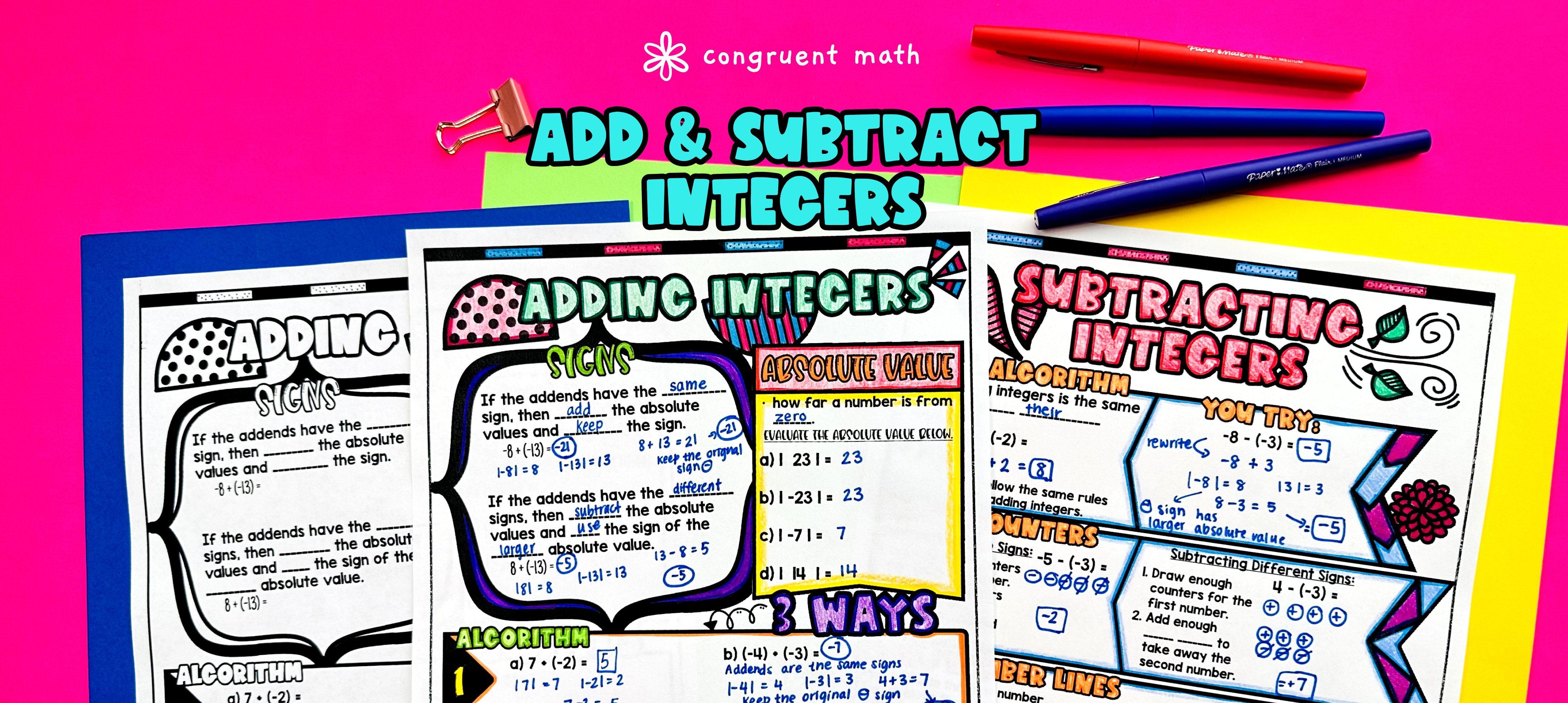Pin This

## Overview

Ever wondered how to teach adding and subtracting integers in an engaging way to your 7th and 8th grade students?

In this lesson plan, students will learn about adding and subtracting integers and their real-life applications. Through artistic, interactive guided notes, check for understanding, a practice color by code activity, and a maze worksheet, students will gain a comprehensive understanding of adding and subtracting integers.

The lesson culminates with a real-life example that explores how this math concept can be applied to tracking scores for games.

## Get the Lesson Materials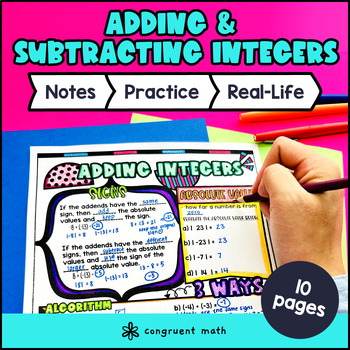\$4.25

## Learning Objectives

After this lesson, students will be able to:

• Add and subtract integers using the algorithm

• Add and subtract integers using counters

• Add and subtract integers using number line diagrams

• Apply the concepts of adding and subtracting integers to solve real-life math problems.

## Prerequisites

Before this lesson, students should be familiar with:

## Key Vocabulary

• Integers

• Algorithm

• Counters

• Number line

• Absolute value

• Zero pairs

## Procedure

### Introduction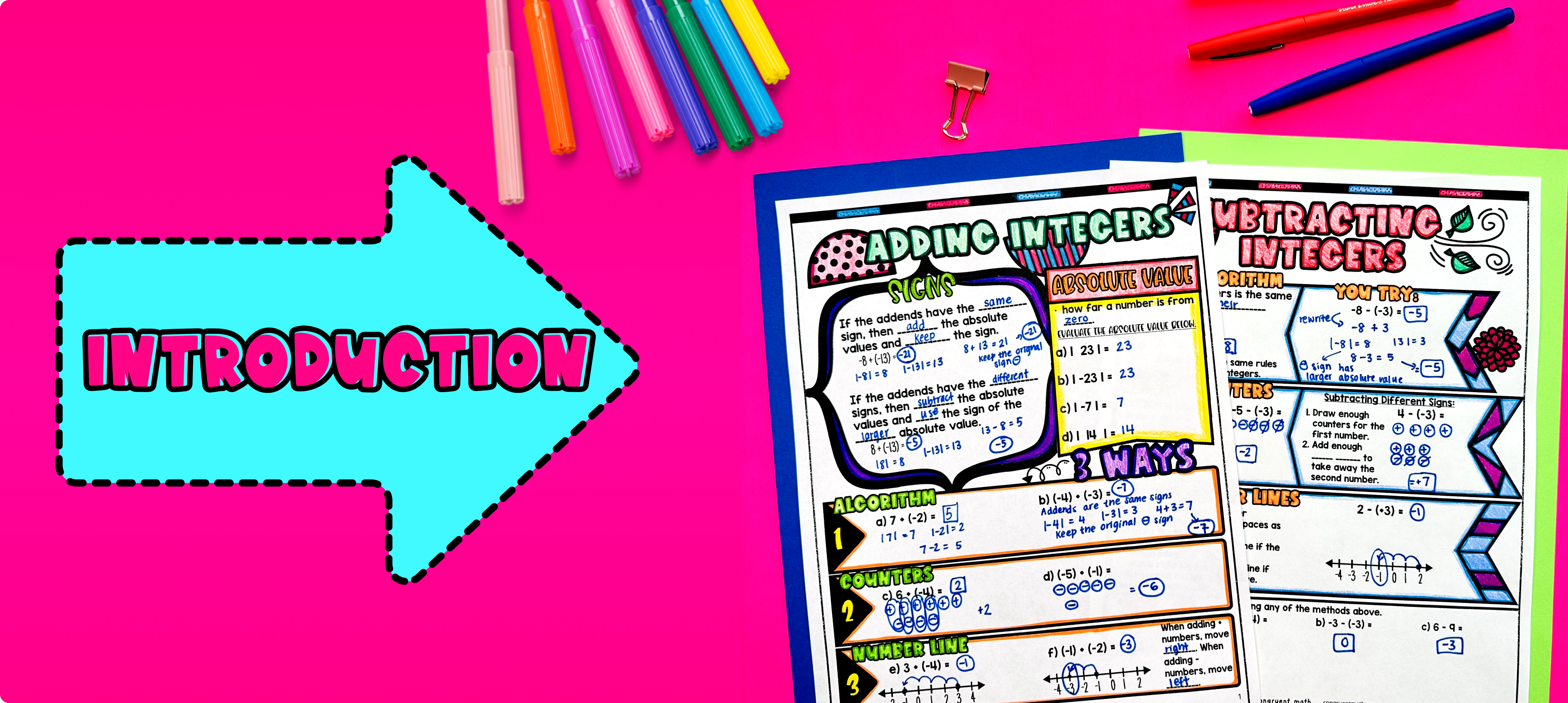Pin This

As a hook, ask students why it is important to understand how to add and subtract integers. Refer to the real-life application of tracking scores for games on the last page of the guided notes as well as the FAQs below for ideas.

Use the first page of the guided notes to introduce the concept of adding integers using counters. Explain to students that they can use counters to visually represent positive and negative numbers and practice adding and subtracting them. Walk through the key points of adding and subtracting integers using counters, such as adding positive and negative numbers, and subtracting positive and negative numbers. Then, introduce the concept of adding integers using number line diagrams and algorithm.

After students have a chance to practice adding integers, pivot to subtracting integers. Using the third page of the guided notes, explain how to subtract integers using the algorithm, counters, and number line diagrams.

Based on student responses, reteach concepts that students need extra help with. If your class has a wide range of proficiency levels, you can pull out students for reteaching, and have more advanced students begin work on the practice exercises.

### Practice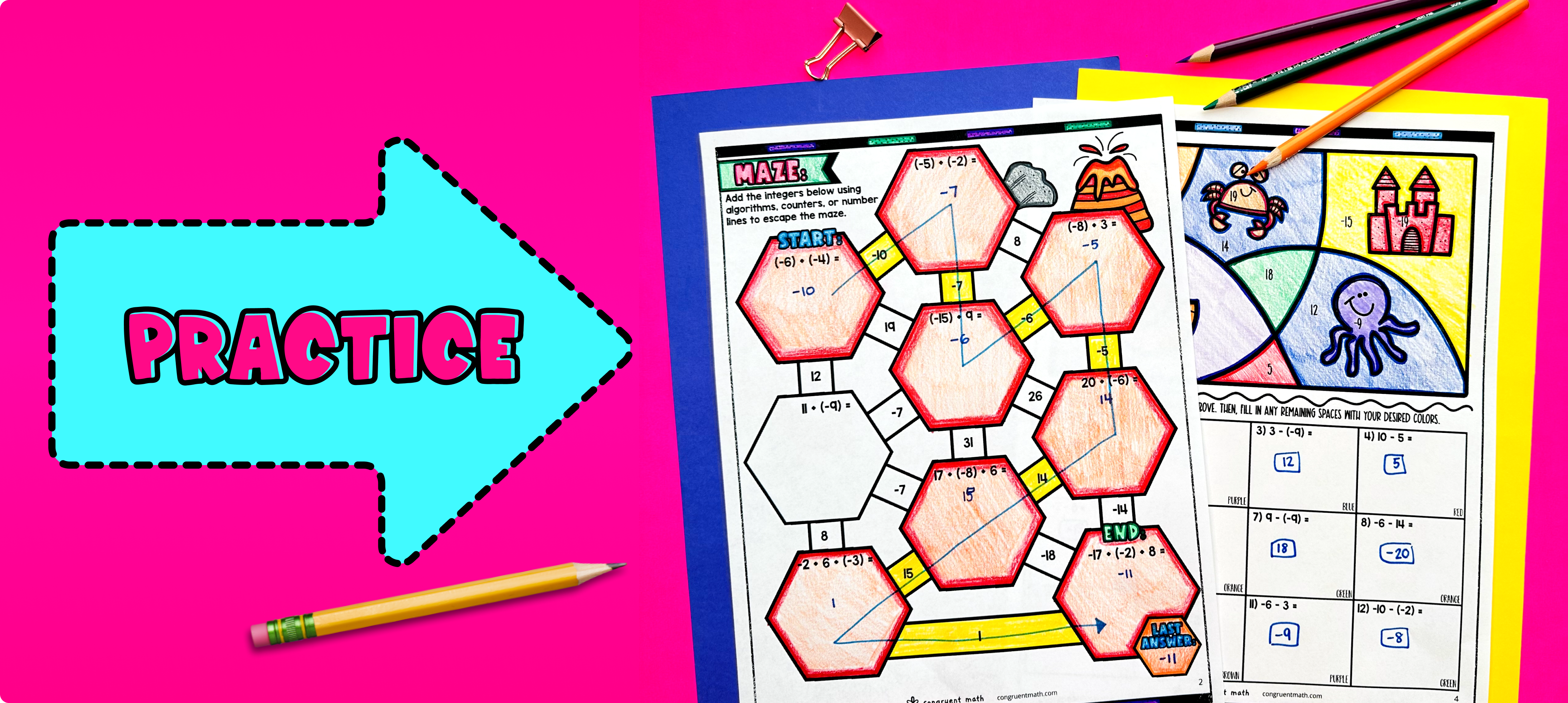Pin This

Have students practice adding and subtracting integers using the practice worksheets provided in the guided notes resource. Walk around the classroom to answer student questions and provide assistance as needed.

You can assign it as homework for the remainder of the class.

### Real-Life Application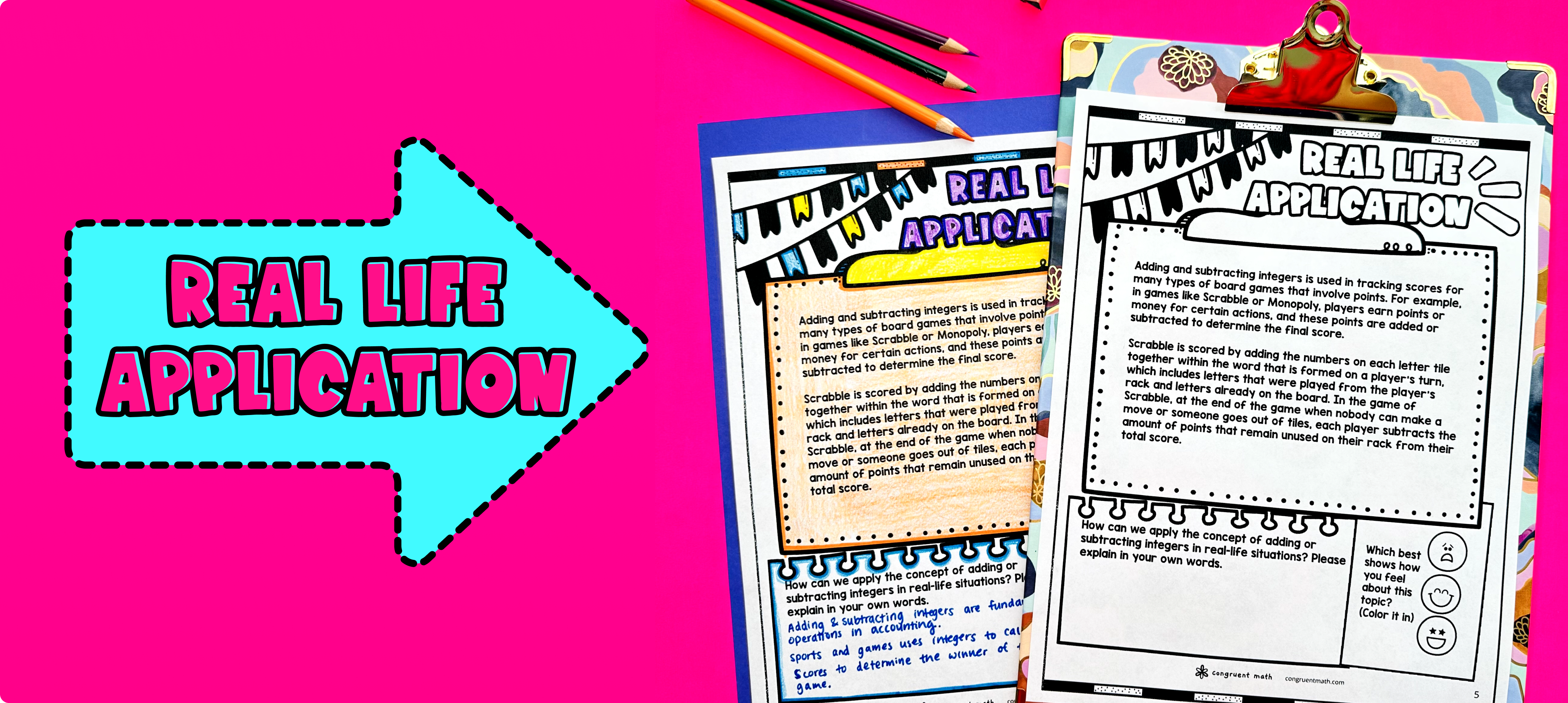Pin This

Bring the class back together, and introduce the concept of tracking scores for games as a real-life application of adding and subtracting integers. Explain to students that when playing games, scores can go up or down depending on performing an action that gains or loses points.

To further illustrate this concept, you can give examples of different games and how scores are tracked. For instance, adding or subtracting integers is used to calculate the player's score based in scrabble or monopoly.

Refer to the real life application section of the guided notes for more ideas on how to incorporate real-world applications into the discussion. Students will then brainstorm some real life application of integers on the last page of the guided notes resource.

## Extensions

If you’re looking for digital practice for adding and subtracting integers, try my Pixel Art activities in Google Sheets. Every answer is automatically checked, and correct answers unlock parts of a mystery picture. It’s incredibly fun, and a powerful tool for differentiation.

Here are some activities to explore: